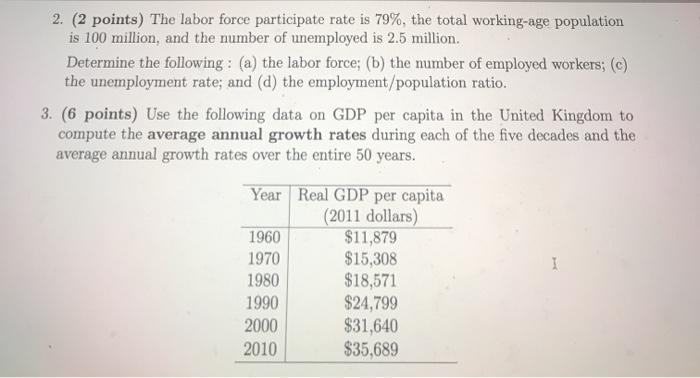### Create an Account

Already have account?

### Forgot Your Password ?

Home / Questions / The labor force participate rate is 79%, the total working-age population is 100 million,...

# The labor force participate rate is 79%, the total working-age population is 100 million, and the number of unemployed is 2.5 million. Determine the following: (a) the labor force; (b) the number of

The labor force participate rate is 79%, the total working-age population is 100 million, and the number of unemployed is 2.5 million. Determine the following: (a) the labor force; (b) the number of employed workers; (c) the unemployment rate; and (d) the employment/population ratio. 3. (6 points) Use the following data on GDP per capita in the United Kingdom to compute the average annual growth rates during each of the five decades and the average annual growth rates over the entire 50 years. I Year Real GDP per capita (2011 dollars) 1960 \$11,879 1970 \$15,308 1980 \$18,571 1990 \$24,799 2000 \$31,640 2010 \$35,689Apr 19 2021 View more View Less

#### Answer (Solved)Subscribe To Get Solution